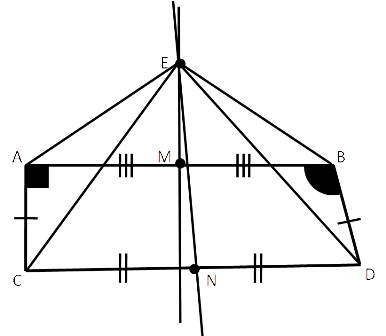A Fallacy in Mathematics is an apparently correct logical argument which leads to an incorrect conclusion.

## Fallacy 1: 1+1=1

Let $a=b$

• $a^2=ab$
• $a^2-b^2=ab-b^2$
• $(a+b)(a-b)=b(a-b)$
• $a+b=b$
• $a+a=a$ (as a=b)
Now let a=1, and hence 1+1=1.

## Fallacy 2: -1 = 1

• $sqrt{-1}=sqrt{-1}$
• $sqrt{\frac{-1}{1}}=sqrt{\frac{1}{-1}}$
• $\frac{sqrt{-1}}{sqrt{1}}=\frac{sqrt{1}}{sqrt{-1}}$
• $sqrt{-1}sqrt{-1}=sqrt{1}{sqrt{1}$
• Hence $-1=1$## Fallacy 3: all angles are right angles

(Rouse Ball's Fallacy)

Construct a quadrilateral ABCD such that AC = BD and angle CAB is a right angle and angle DBA is obtuse.

• AB is therefore not parallel to CD,
• hence perpendicular bisectors are not parallel
• hence perpendicular bisectors intersect at some point: call it E
• Construct the perpendicular bisector of AB namely ME
• Construct the perpendicular bisector of CD namely NE
• Triangle AEM is congruent to triangle BEM (RHS)
• thus angle EAM = angle EBM
• Triangle ACE is congruent to triangle BDE (SSS)
• thus angle EAC = angle EBD
• Subtracting these angles gives angle CAB = angle DBA
Therefore all obtuse angles are right angles.

Similarly all acute angles are right angles (complement of obtuse angles) ... all angles are right angles.